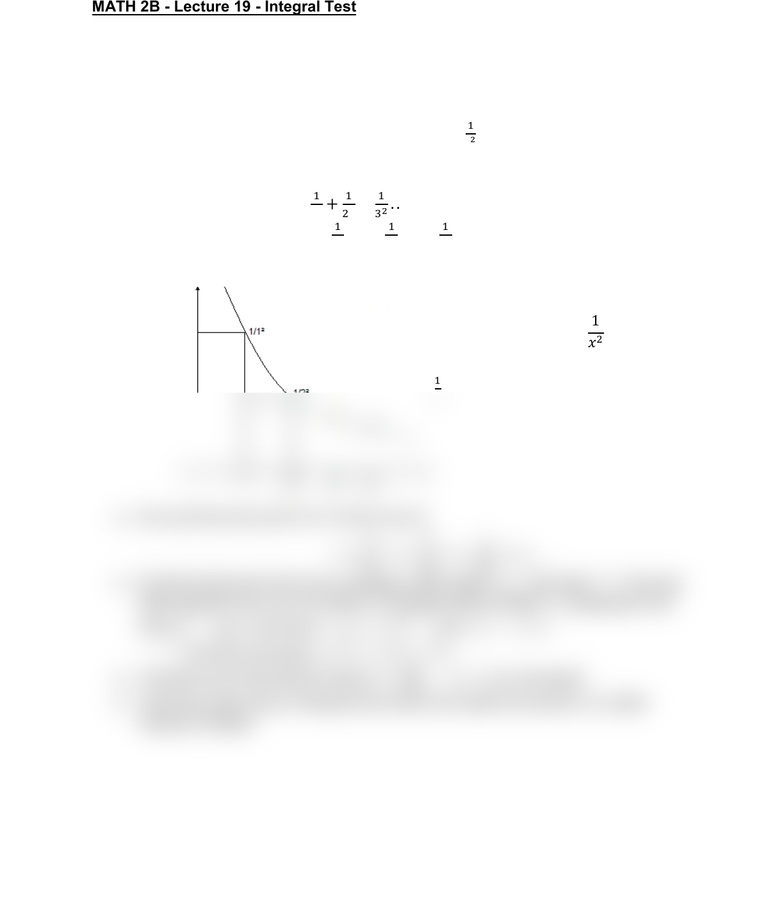Class Notes (1,100,000)
US (460,000)
UC-Irvine (10,000)
MATH (1,000)
MATH 2B (600)
Lecture 20

# MATH 2B Lecture 20: Integral TestPremium

Department
Mathematics
Course Code
MATH 2B
Professor
ERJAEE, G.
Lecture
20

This preview shows half of the first page. to view the full 2 pages of the document.02/20/2019
MATH 2B - Lecture 19 - Integral Test
For most of the series, we are not able to find , therefore we need other
techniques for convergence test
Example:
Determine whether the series

is convergent
Solution:
Series  
Multiply by 1:  

Recall the definition of improper integral

We can find every term in S on the curve
We then treat each term as a rectangle, with height , and base “1”. If we use
right-endpoint rule, we can obtain a rectangle approximation. Comparing to the
area
, we know

Also we know that  
Therefore we know that the series
is convergent
That’s the basic idea of integral test, where we relate the series to a certain
improper integral